# Resistance Temperature Detectors Working Principle

Resistance Temperature Detectors (RTD) Working Principle

Thermo-resistive Temperature Measuring Devices

A change in temperature causes the electrical resistance of a material to change. The resistance change is measured to infer the temperature change.

There are two types of thermo-resistive measuring devices:

• Resistance temperature detectors (RTD) and
• Thermistors

Resistance Temperature Detectors

A resistance temperature detector (abbreviated RTD) is basically either a long, small diameter metal wire wound in a coil or an etched grid on a substrate, much like a strain gage. Platinum is the most common metal used for RTDs.

Principle of Operation

Resistance Temperature Detectors (RTD) operates on the principle that the electrical resistance of a metal changes predictably in an essentially linear and repeatable manner with changes in temperature. RTD have a positive temperature coefficient (resistance increases with temperature). The resistance of the element at a base temperature is proportional to the length of the element and the inverse of the cross sectional area.

A typical electrical circuit designed to measure temperature with RTDs actually measures a change in resistance of the RTD, which is then used to calculate a change in temperature. The resistance of an RTD increases with increasing temperature, just as the resistance of a strain gage increases with increasing strain.

Bridge Circuit Construction

Figure below shows a basic bridge circuit which consists of three known resistances, R1, R2, and R3 (variable), an unknown variable resistor RX (RTD), a source of voltage, and a sensitive ammeter.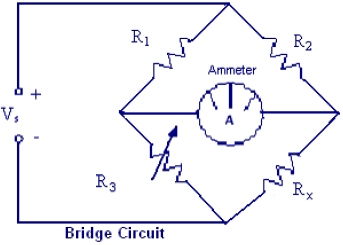Resistors R1 and R2 are the ratio arms of the bridge. They ratio the two variable resistances for current flow through the ammeter. R3 is a variable resistor known as the standard arm that is adjusted to match the unknown resistor. The sensing ammeter visually displays the current that is flowing through the bridge circuit. Analysis of the circuit shows that when R3 is adjusted so that the ammeter reads zero current, the resistance of both arms of the bridge circuit is the same. The relationship of the resistance between the two arms of the bridge can be expressed asSince the values of R1, R2, and R3 are known values, the only unknown is Rx. The value of Rx can be calculated for the bridge during an ammeter zero current condition. Knowing this resistance value provides a baseline point for calibration of the instrument attached to the bridge circuit. The unknown resistance, Rx, is given by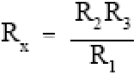RTD Bridge Circuit Operation

One simple circuit is the quarter bridge Wheatstone bridge circuit, here called a two-wire RTD bridge circuit.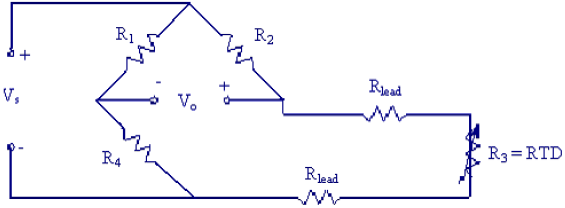Rlead represents the resistance of one of the wires (called lead wires) that run from the bridge to the RTD itself. Lead resistance was of no concern in strain gage circuits because Rlead remained constant at all times.

For RTD circuits, however, some portions of the lead wires are exposed to changing temperatures. Since the resistance of metal wire changes with temperature, R lead changes with T, which can cause errors in the measurement. This error can be non-trivial – changes in lead resistance may be misinterpreted as changes in RTD resistance. Furthermore, there are two lead wires in the two-wire RTD bridge circuit shown above, which doubles the error. A clever circuit designed to eliminate the lead wire resistance error is called a three-wire RTD bridge circuit. The three-wire RTD bridge circuit is shown below.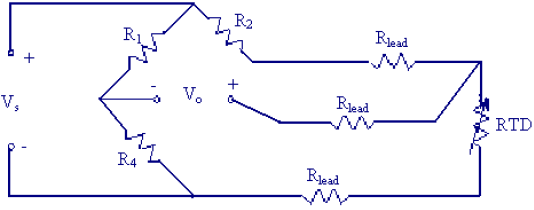It is still a quarter bridge circuit, since only one of the four bridge resistors has been replaced by the RTD. However, one of the lead wires has been placed on the R2 leg of the bridge instead of the R3 leg.

To analyze this circuit, assume that R1 = R4, and R2 = R3 initially, when the bridge is balanced.Recall the general formula for a Wheatstone bridge: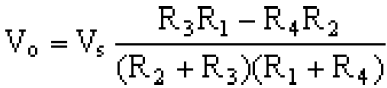Notice that R3 and R2 have opposite signs in the above equation. So, if the lead wire resistance in leg 2 (top) and that in leg 3 (bottom) are the same, the lead resistances cancel each other out, with no net effect on the output voltage, thus eliminating the error.

What about the third lead resistance, Rlead of the middle wire? Well, since Vo is measured with a nearly infinite impedance device, no current flows in the middle lead wire, so its resistance does not affect anything!

The following re-drawn equivalent circuit may help explain why the lead resistances cancel out: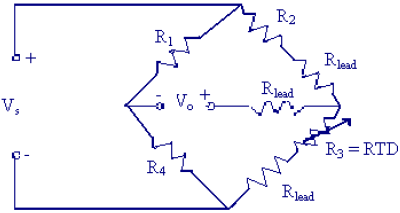In the above diagram, it is clear that if Rlead changes equally in leg 2 and leg 3 of the bridge, its effect cancels out.

RTD Materials & Construction

RTD acts somewhat like an electrical transducer, converting changes in temperature to voltage signals by the measurement of resistance. The metals that are best suited for use as RTD sensors are pure metals or certain alloys of uniform quality that increase in resistance as temperature increases and conversely decrease in resistance as temperature decreases. Only a few metals have the properties necessary for use in RTD elements. Common materials used in RTD sensor are BALCO wire, Copper, Platinum.

• BALCO – A sensor constructed using a BALCO wire is an annealed resistance alloy with a nominal composition of 70 percent nickel and 30 percent iron. A BALCO 500-ohm resistance element provides a relatively linear resistance variation from –40 to 250° The sensor is a low-mass device and responds quickly to changes in temperature. When 1000 ohms is measured across the BALCO element, the temperature is approximately 70°F. As the temperature increases, the resistance changes 2.2 ohms per 1°F. This is called a Temperature Coefficient of Resistance Curve (TCR Curve). In a BALCO, as the resistance has direct relationship with temperature i.e. as temperature increases, the resistance increases proportionally. The usual range of temperature measurement with BALCO is -40° to 240°F.
• Platinum – RTD sensors using platinum material exhibit linear response and stable over time. In some applications a short length of wire is used to provide a nominal resistance of 100 ohms. However, with a low resistance value, element self-heating and sensor lead wire resistance can effect the temperature indication. With a small amount of resistance change of the element, additional amplification must be used to increase the signal level. Platinum film sensor on an insulating base provides high resistance to the tune of 1000 ohms at 74° With this high resistance, the sensor is relatively immune to self-heating and responds quickly to changes in temperature. RTD elements of this type are common.

These metals are best suited for RTD applications because of their linear resistance-temperature characteristics (as shown in figure below), their high coefficient of resistance, and their ability to withstand repeated temperature cycles. The coefficient of resistance is the change in resistance per degree change in temperature, usually expressed as a percentage per degree of temperature. The material used must be capable of being drawn into fine wire so that the element can be easily constructed.Copper and nickel versions operate at lower temperature ranges and are less expensive than platinum. Platinum is the most versatile material because of its wide temperature range (–200°C to 850°C), excellent repeatability, stability, and resistance to chemicals and corrosion.RTD elements are usually long, spring- like wires surrounded by an insulator and enclosed in a sheath of metal. Figure below shows the internal construction of an RTD.

This particular design has a platinum element that is surrounded by a porcelain insulator. The insulator prevents a short circuit between the wire and the metal sheath. Inconel, a nickel-iron-chromium alloy, is normally used in manufacturing the RTD sheath because of its inherent corrosion resistance. When placed in a liquid or gas medium, the Inconel sheath quickly reaches the temperature of the medium. The change in temperature will cause the platinum wire to heat or cool, resulting in a proportional change in resistance.

Advantages: Linear resistance with temperature, good stability, wide range of operating temperature Interchangeable over wide temperature range

Disadvantages: Small resistance change with temperature, responses may be slower, subject to self heating, transmitter or three to four wire leads required for lead resistance compensation, external circuit power required

• RTD’s are commonly used in sensing air and liquid temperatures in pipes and ducts, and as room temperature sensors. The resistance of RTD elements varies as a function of temperature. Some elements exhibit large resistance changes, linear changes, or both over wide temperature ranges.
• Varying voltage across the sensor element determines the resistance of the sensor. The power supplied for this purpose can cause the element to heat slightly and can create an inaccuracy in the temperature measurement. Reducing supply current or by using elements with higher nominal resistance can minimize the self-heating effect.
• Some RTD element resistances are as low as 100 ohms. In these cases, the resistance of the lead wires connecting the RTD to the controller may add significantly to the total resistance of the connected RTD, and can create an error in the measurement of the temperature. For instance, a sensor placed 25 feet from the controller has a copper control wire of 25 x 2 = 50 feet. If a control wire has a DC resistance of 6.39 ohms/ft, the 50 feet of wire shall have a total dc resistance of 0.319 ohms. If the sensor is a 100-ohm platinum sensor with a temperature coefficient of 0.69 ohms per degree F, the 50 feet of wire will introduce an error of 0.46 degrees F. If the sensor is a 3000-ohm platinum sensor with a temperature coefficient of 4.8 ohms per degree F, the 50 feet of wire will introduce an error of 0.066 degrees F.

Therefore lesser is the resistance of sensor element, higher shall be the likelihood of error. Significant errors can be removed by adjusting a calibration setting on the controller, or, if the controller is designed for it, a third wire can be run to the sensor and connected to a special compensating circuit designed to remove the lead length effect on the measurement.

Also Read: Thermocouple Working Principle

Don't Miss Our Updates
Be the first to get exclusive content straight to your email.
We promise not to spam you. You can unsubscribe at any time.

### 10 thoughts on “Resistance Temperature Detectors Working Principle”

1.Dear Sir,

1. How can we convert ohms into degree celsius (temperature) for RTD ?
2. How can we convert millivolt into degree celsius (temperature) for Thermocouple?

Thank You.

•For RTD ohms to temperature conversion formula: click Here
For Thermocouple millivolt to temperature conversion Formula: Click Here

2.Sir, What is the use of 3rd wire in 3 wire RTD. Please explain me clearly. Also explain me about 4 wire.

3.Thank you very much sir….detailed description about RTD..

4.How to convert 2 wire RTD in to 3 wire and vice versa??

5.Is there really no effect of the lead resistance. Do they really fully cancel out?

•Of Course Yes, it depends on type of RTD we are using. Click Here to See the differences.

6.Lead resistance of R2 and R3 will cancel each other in the nominator but in the denominator there is a summation of R2 and R3 which will not be canceled

7.Quoting:
For instance, a sensor placed 25 feet from the controller has a copper control wire of 25 x 2 = 50 feet. If a control wire has a DC resistance of 6.39 ohms/ft, the 50 feet of wire shall have a total dc resistance of 0.319 ohms.

Question:
How is this so? Is not the RTD and its leads a series circuit such that 6.39 ohms/ft X 50 ft. would yield 319.5 ohms? This value would ADD to the resistance of the sensor itself, and would need to be balanced out of the circuit to remove the offset induced by the leads.

If I am correct, then the error induced by these particular leads would be much greater. Was it intended that the lead resistance should be 0.00693 ohms/ft., possibly? That must be it! 50 ft. of 18 ga. AWG copper wire would have a total resistance of 0.319 ohms per standard reference tables.

•